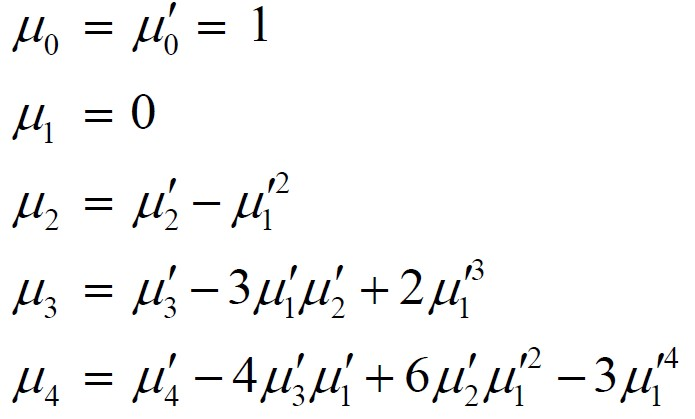# ML | Raw and Central Moments

Moments are a set of statistical parameters which are used to describe different characteristics and feature of a frequency distribution i.e. central tendency, dispersion, symmetry, and peakedness (hump) of the frequency curve. For Ungrouped data i.e. discrete data, observations on a variable X are obtained as, For Grouped data i.e. continuous data, observations on a variable X are obtained and tabulated in K class intervals in a frequency table. The mid points of the intervals are denoted bywhich occur with frequenciesrespectively and.

Moments about an arbitrary point A Themoment of a variable X about any arbitrary point A on the observationsis defined as:

For ungrouped dataFor grouped datawhereMoment about any arbitrary point in Python – Consider the given data points. Following are the time (in hours) spent by 20 different persons at GeeksforGeeks portal every week.

15, 25, 18, 36, 40, 28, 30, 32, 23, 22, 21, 27, 31, 20, 14, 10, 33, 11, 7, 13

## Python3

 # data points time = [15, 25, 18, 36, 40, 28, 30, 32, 23, 22,          21, 27, 31, 20, 14, 10, 33, 11, 7, 13]   # Arbitrary point  A = 22   # Moment for r = 1 moment = (sum([(item-A) for item in time]))/len(time)

## Raw Moments –

Themoment around origin A = 0 known as raw moment and is defined as:

For ungrouped data,For grouped data,where,Notes:

-> We can find first raw moment () just by replacing r with 1 and second raw moment () just by replacing r with 2 and so on. -> When r = 0 the momentfor both grouped and ungrouped data.

Raw moment in Python –

## Python3

 # data points time = [15, 25, 18, 36, 40, 28, 30, 32, 23,        22, 21, 27, 31, 20, 14, 10, 33, 11, 7, 13]     # Moment for r = 1 moment = sum(time)/len(time)

## Central Moments –

The moments of a variable X about the arithmetic mean () are known as central moments and defined as:

For ungrouped data,For grouped data,whereandNotes:

-> We can find first raw moment () just by replacing r with 1 and second raw moment () just by replacing r with 2 and so on. -> When r = 0 the moment, and when r = 1 the momentfor both grouped and ungrouped data.

## Python3

 # data points time = [15, 25, 18, 36, 40, 28, 30, 32, 23, 22,        21, 27, 31, 20, 14, 10, 33, 11, 7, 13]   # Mean  A = sum(time)/len(time)   # Moment for r = 1 moment = (sum([(item-A) for item in time]))/len(time)

Relationship between Raw and Central moments –Whether you're preparing for your first job interview or aiming to upskill in this ever-evolving tech landscape, GeeksforGeeks Courses are your key to success. We provide top-quality content at affordable prices, all geared towards accelerating your growth in a time-bound manner. Join the millions we've already empowered, and we're here to do the same for you. Don't miss out - check it out now!

Previous
Next